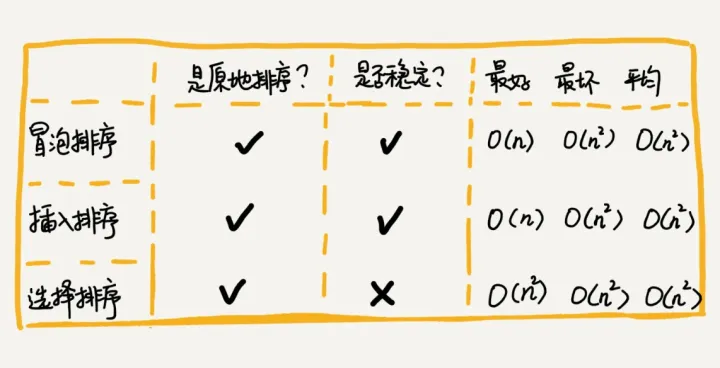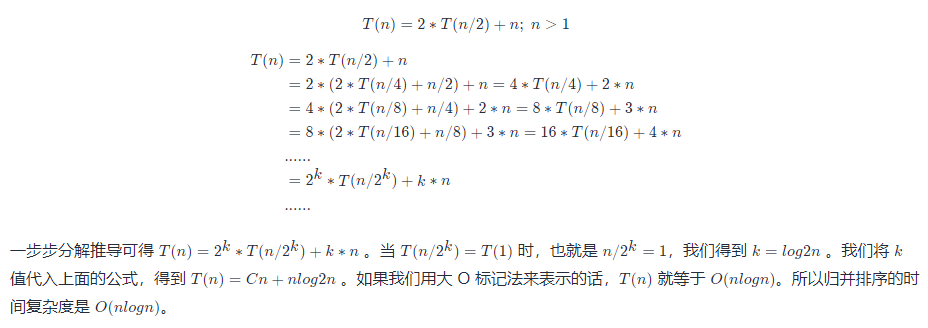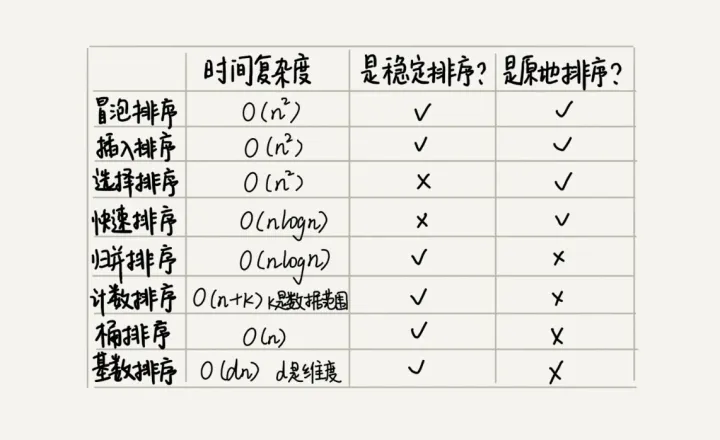## 一，冒泡排序（Bubble Sort）

``````// 将数据从小到大排序
void bubbleSort(int array[], int n){
if (n<=1) return;
for(int i=0; i<n; i++){
for(int j=0; j<n-i; j++){
if (temp > a[j+1]){
temp = array[j]
a[j] = a[j+1];
a[j+1] = temp;
}
}
}
}``````

``````// 将数据从小到大排序
void bubbleSort(int array[], int n){
if (n<=1) return;
for(int i=0; i<n; i++){
// 提前退出冒泡循环发标志位
bool flag = False;
for(int j=0; j<n-i; j++){
if (temp > a[j+1]){
temp = array[j]
a[j] = a[j+1];
a[j+1] = temp;
flag = True; // 表示本次冒泡操作存在数据交换
}
}
if(!flag) break; // 没有数据交换，提交退出
}
}``````

1. 冒泡过程只涉及相邻元素的交换，只需要常量级的临时空间，故空间复杂度为 O(1)O(1)，是原地排序算法
2. 当有相邻的两个元素大小相等的时候，我们不做交换，相同大小的数据在排序前后不会改变顺序，所以是稳定排序算法
3. 最坏情况和平均时间复杂度都为 O(n2)O(n2)，最好时间复杂度是 O(n)O(n)。

## 二，插入排序（Insertion Sort）

1. 插入排序算法将数组中的数据分为两个区间：已排序区间和未排序区间。最初始的已排序区间只有一个元素，就是数组的第一个元素。
2. 插入排序算法的核心思想就是取未排序区间的一个元素，在已排序区间中找到一个合适的位置插入，并保证已排序区间数据一直有序。
3. 重复这个过程，直到未排序区间元素为空，则算法结束。

``````void InsertSort(int a[], int n){
if (n <= 1) return;
for (int i = 1; i < n; i++) // 未排序区间范围
{
key  = a[i]; // 待排序第一个元素
int j = i - 1; // 已排序区间末尾元素
// 从尾到头查找插入点方法
while(key < a[j] && j >= 0){ // 元素比较
a[j+1] = a[j]; // 数据向后移动一位
j--;
}
a[j+1] = key; // 插入数据
}
}``````

1. 插入排序并不需要额外存储空间，空间复杂度是 O(1)O(1)，所以插入排序也是一个原地排序算法。
2. 在插入排序中，对于值相同的元素，我们可以选择将后面出现的元素，插入到前面出现元素的后面，这样就可以保持原有的前后顺序不变，所以插入排序是稳定的排序算法。
3. 最坏情况和平均时间复杂度都为 O(n2)O(n2)，最好时间复杂度是 O(n)O(n)。

## 三，选择排序（Selection Sort）

``````void SelectSort(int a[], int n){
for(int i=0; i<n; i++){
int minIndex = i;
for(int j = i;j<n;j++){
if (a[j] < a[minIndex]) minIndex = j;
}
if (minIndex != i){
temp = a[i];
a[i] = a[minIndex];
a[minIndex] = temp;
}
}
}``````

### 冒泡插入选择排序总结## 四，归并排序（Merge Sort）

``````递推公式：
merge_sort(p…r) = merge(merge_sort(p…q), merge_sort(q+1…r))

p >= r 不用再继续分解，即区间数组元素为 1 ``````

``````merge_sort(A, n){
merge_sort_c(A, 0, n-1)
}
merge_sort_c(A, p, r){
// 递归终止条件
if (p>=r) then return
// 取 p、r 中间的位置为 q
q = (p+r)/2
// 分治递归
merge_sort_c(A[p, q], p, q)
merge_sort_c(A[q+1, r], q+1, r)
// 将A[p...q]和A[q+1...r]合并为A[p...r]
merge(A[p...r], A[p...q], A[q+1...r])
}``````

### 4.1，归并排序性能分析

1，归并排序是一个稳定的排序算法。分析：伪代码中 merge_sort_c() 函数只是分解问题并没有涉及移动元素和比较大小，真正的元素比较和数据移动在 merge() 函数部分。在合并过程中保证值相同的元素合并前后的顺序不变，归并排序排序就是一个稳定的排序算法。

2，归并排序的执行效率与要排序的原始数组的有序程度无关，所以其时间复杂度是非常稳定的，不管是最好情况、最坏情况，还是平均情况，时间复杂度都是 O(nlogn)O(nlogn)。分析：不仅递归求解的问题可以写成递推公式，递归代码的时间复杂度也可以写成递推公式：3，空间复杂度是 O(n)。分析：递归代码的空间复杂度并不能像时间复杂度那样累加。尽管算法的每次合并操作都需要申请额外的内存空间，但在合并完成之后，临时开辟的内存空间就被释放掉了。在任意时刻，CPU 只会有一个函数在执行，也就只会有一个临时的内存空间在使用。临时内存空间最大也不会超过 n 个数据的大小，所以空间复杂度是 O(n)O(n)。

## 五，快速排序（Quicksort）

``````递推公式：
quick_sort(p,r) = quick_sort(p, q-1) + quick_sort(q, r)

p >= r``````

### 归并排序和快速排序总结## 参考资料

• 排序（上）：为什么插入排序比冒泡排序更受欢迎？
• 排序（下）：如何用快排思想在O(n)内查找第K大元素？